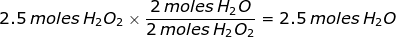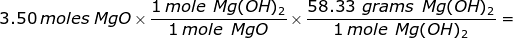# Theoretical Yield Formula

Theoretical Yield Formula

In a chemical reaction the maximum amount of product formed is determined by the amount of limiting reactant that is used up. Stoichiometry is used to predict this amount of product. It is known as the theoretical yield.

Theoretical Yield Formula Questions:

1. Determine the theoretical yield of H2O (in moles) in the following reaction, if 2.5 moles of hydrogen peroxide are decomposed.

2H2O2 → 2H2O + O2

In this reaction there is only one reactant (H2O2) so it must be the limiting reactant. Stoichiometry will be used to determine the moles of water that can be formed.2. How many grams of magnesium hydroxide can be formed from the reaction of 3.50 moles of magnesium oxide with an excess of water?

MgO + H2O → Mg(OH)2

The problem states that water is the excess reactant which means that magnesium oxide is limiting. Stoichiometry will be used to determine the grams of magnesium hydroxide that can be created from 3.50 moles of magnesium oxide. The molar mass of magnesium hydroxide must be determined in order to convert from moles of magnesium hydroxide to grams.204 grams Mg(OH)2

 Related Links: Emmy Noether Facts Arnold Sommerfeld Facts Stephen Hawking Facts Stoichiometry V: Percent Yield Quiz